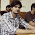## Wednesday, February 27, 2013

### Trick to multiply three digit number with 11 verbally?

It is the extension of the trick that we done in last post  check it
It is easy to multiply three digit with 11 verbally.
Consider the number that we want to multiply with 11 For e.g. 123

123* 11 =           1353

123
*11
1 3  5 3

The first digit from the right hand side will be the first digit of the number we are multiplying with 11

Here it  3 from 123

Second digit will be the sum of   the first two digit of the number here it is  3 + 2 = 5

Third digit  will be the sum of last two digit of the number here it is 2 + 1 = 3

last digit will be the last digit of the number here it  is 1

So answer is 1353

Note:- Add carry from all these addition to next number
Check this E.g.

Multiplication of number 999 by 11

999
× 11
10 989

first digit = 9
Second digit = 9+9 = 18 so the number is  8 carry 1
third  digit  =  9+9  18 plus the carry + 1 equal to 19
fourth digit = 9 + 1 equal to 10

10989

Now calculate the value of  571×11, 589 ×11 and post

Hope you like this.

1.amazing trick.

2.571*11=6281.
this trick works.

3.4.great work